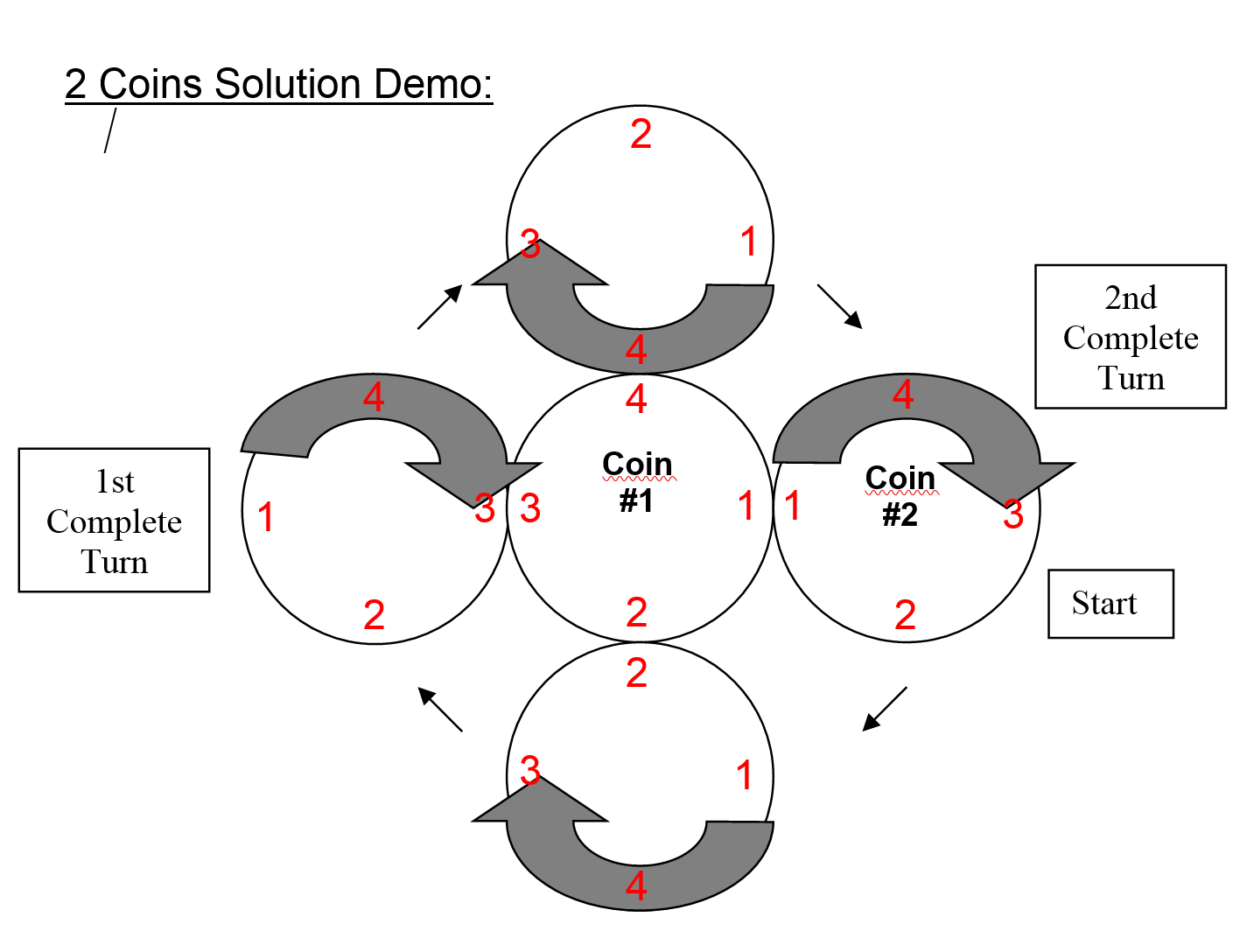Select PagePrintable version

Puzzle one

Solve the following equation if N, O & G are single digit numbers and NO & GN are double digit numbers.  Find the values for N, O & G.### Solution

learn_more caption=”Solution explanation”]

1. N can only be equal to either 1 or 2; otherwise G is a two digit number. (i.e., N + N + N + N = G or N x 4 = G)
2. N has to be an even number (i.e., O + O + O + O = N or O x 4 = N)! Therefore, N = 2, since 4 times any number will always result in an even number.
3. Since O is still unknown, G (G = N x 4) can only be equal to either 8 or 9;  8 if there is no carry over from the Equation O x 4 = N (where N x 4 = G or = 8), or 9 if there is a carry over from the Equation O x 4 = N (where (N x 4) +1 = G or = 9).
4. Solving for O without a carry over:
• ((Nx10) + O) x 4 = 82
• (20 + O) x 4 = 82
• 80 + (4 x O) = 82
• 4 x O = 2
• O = 0.5 (not valid, must be a whole number)
5. Solving for O with a carry over:
• [((Nx10) + O) x 4] = 92
• (20 + O) x 4 = 92
• 80 + (4 x O) = 92
• 4 x O = 12
• O = 3 (valid answer)
6. Now check the solution:
• NO + NO + NO + NO = GN
• 23 + 23 + 23 + 23 = 92

Puzzle two

A ball travels a certain distant before bouncing, half as far before the next bounce, and so on.  If it has traveled 93 meters at the fifth bounce, how far was the first bounce?48 meters

### Solution explanation

We know the ball bounces five times in 93 meters.  We also know that the ball bounces half the distance each time.  Working backwards, we can write this as:

93 = 1x + 2x + 4x + 8x + 16x

= 31x

Therefore x = 3 and the first bounce was 16 x 3 = 48 meters

Puzzle three

With Gear #1 stationary, how many complete turns does the right gear (#2) make to go around the left gear (#1) once?### Solution

Gear #2 turns twice.## Feedback

There are more than one way of doing these puzzles and may well be more than one answer.  Please let me and others know what alternatives you find by commenting below.  We also welcome general comments on the subject and any feedback you’d like to give.   If you have a question that need a response from me or you would like to contact me privately, please use the contact form.

## Get more puzzles!

If you’ve enjoyed doing the puzzles, consider ordering the book; 150+ of the best puzzles in a handy pocket sized format. Click here for full details.

### Share This

Share this post with your friends!